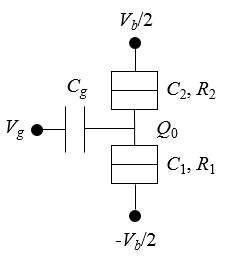## Symmetrically biased single-electron transistor

 A single electron transistor consisting of a conducting island coupled to two tunnel junctions and a gate capacitor is biased symmetrically. The tunnel junctions have capacitances $C_1$, $C_2$ and resistances $R_1$, $R_2$. The gate capacitance is $C_g$ and the offset charge on the island is $Q_0$. A bias voltage of $-V_b/2$ is applied to tunnel junction 1 and a bias voltage $V_b/2$ is applied to tunnel junction 2. A gate voltage $V_g$ is applied to the gate. The form below can be used to plot the current voltage characteristics for bias voltages at temperature $T$ from $V_b$(start) to $V_b$ (stop). $N$ is the number of points used in the plot. The equations that are used are described in Günther Lientschnig, Irek Weymann and Peter Hadley, Simulating Hybrid Circuits of Single-Electron Transistors and Field-EffectTransistors, Japanese Journal of Applied Physics 42 pp. 6467-6472 (2003).$I$ [nA] $V_b$ [mV]
 $C_1$ = F $C_2$ = F $R_1$ = $\Omega$ $R_2$ = $\Omega$ $C_{g}$ = F $V_{g}$ = V $Q_0$ = $e$ $T$ = K $V_b$ (max) = V $N$ =

 $V_b$ [V] $I$ [A]